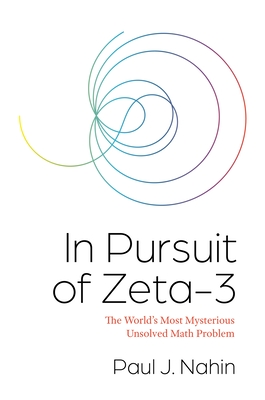# In Pursuit of Zeta-3: The World's Most Mysterious Unsolved Math Problem

Current price: \$26.95
Publication Date: October 19th, 2021
Publisher:
Princeton University Press
ISBN:
9780691206073
Pages:
344
The MIT Press Bookstore
2 on hand, as of Dec 8 10:45am
(MATH)
On Our Shelves Now

## Description

An engrossing look at the history and importance of a centuries-old but still unanswered math problem

For centuries, mathematicians the world over have tried, and failed, to solve the zeta-3 problem. Math genius Leonhard Euler attempted it in the 1700s and came up short. The straightforward puzzle considers if there exists a simple symbolic formula for the following: 1+(1/2) 3+(1/3) 3+(1/4) 3+. . . . But why is this issue--the sum of the reciprocals of the positive integers cubed--so important? With In Pursuit of Zeta-3, popular math writer Paul Nahin investigates the history and significance of this mathematical conundrum.

Drawing on detailed examples, historical anecdotes, and even occasionally poetry, Nahin sheds light on the richness of the nature of zeta-3. He shows its intimate connections to the Riemann hypothesis, another mathematical mystery that has stumped mathematicians for nearly two centuries. He looks at its links with Euler's achievements and explores the modern research area of Euler sums, where zeta-3 occurs frequently. An exact solution to the zeta-3 question wouldn't simply satisfy pure mathematical interest: it would have critical ramifications for applications in physics and engineering, such as quantum electrodynamics. Challenge problems with detailed solutions and MATLAB code are included at the end of each of the book's sections.

Detailing the trials and tribulations of mathematicians who have approached one of the field's great unsolved riddles, In Pursuit of Zeta-3 will tantalize curious math enthusiasts everywhere.